# A Guide to Principal Component Analysis

## Introduction

Machine learning deals with lots of data. Oftentimes, we work with many variables, which can lead to issues regarding the efficiency of the program. One helpful technique to mitigate this problem is dimensionality reduction. Somehow, we try to reduce the number of input variables while trying our best not to compromise the results of the program. This reduces the amount of necessary storage space and reduces the computation time . In this article, I will give a run-down on one algorithm commonly used in dimensionality reduction — Principal Component Analysis (PCA).

## Background Math

Most basic linear algebra classes deal with the concept of dimensionality. You might think of it as the space ℝ, ℝ², or even ℝⁿ to represent n-dimensions. While dealing with data, we like to think of dimensionality as the number of input variables. This makes sense when we correlate with linear algebra — one input variable can scale across the x-axis, two variables give the x-y coordinate system, three provide the x-y-z coordinate system, and so on. In dimensionality reduction, we project the original dataset from n dimensions into a new space of m dimensions, where m < n.

## Normalizing the Data

Linear algebra (and math in general) is a lot simpler when the number zero is involved! Therefore, the first step in PCA is to center our data around the zero vector, acheived by subtracting the mean of the dataset from every datapoint (x_new = x_old-μ). This “shifts” the whole set to be centered around zero. Our next step is to ensure that measurement biases do not affect the process of PCA. This is done by dividing every datapoint by its standard deviation. The entire process detailed in this paragraph is called normalization, and the final formula stands: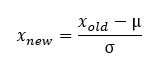The process of normalization, where x_new is the normalized vector of x_old.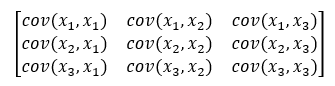Building a covariance matrix.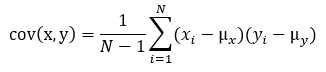The covariance between two variables.

## Making Sense of the Covariance Matrix

The eigenvalues and eigenvectors of the covariance matrix are important. Since the covariance matrix is symmetric, from the spectral theorem, we can diagonalize this covariance matrix into the form P¹DP, where P is the matrix formed by using the eigenvectors as row vectors, and D is a diagonal matrix where every entry is the corresponding eigenvalue . The eigenvectors of the covariance matrix show the direction of the variables’ spread — all the eigenvectors must be orthogonal to each other. The eigenvalues refer to the magnitude. They answer the question: How much do these variables spread in this particular direction (eigenvector)?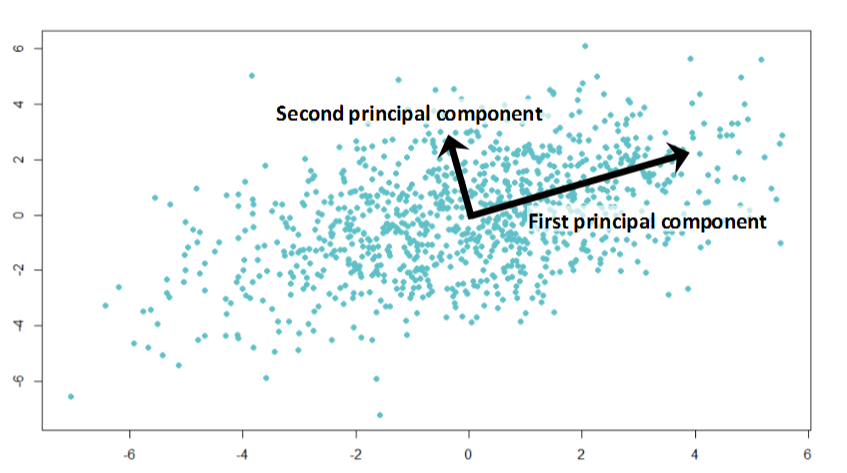The diagram above shows a set of data with two variables. The first principal component follows the direction of maximum variance, and the second principal component is the direction of maximum variance that is orthogonal to the first. (Source: Analytics Vidhya)

## Principal Components

The most important principal component stores the most information. The least important stores the least information. Thus, we finally get to the objective of PCA: Discard the least important principal components (we will undoubtedly lose some information unless we live in an ideal world where some eigenvalues are zero) and keep the remaining ones, so we can reduce the dimensionality of the original dataset. How many eigenvectors to discard is up to the programmer — we are sacrificing accuracy for efficiency here. The original dataset is projected onto the new axes formed by the chosen principal components, and further machine learning algorithms are performed on these new axes. The amount of information loss by sacrificing any given eigenvector can be calculated with the formula: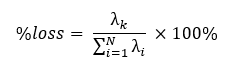The loss of information due to PCA compared to the original information.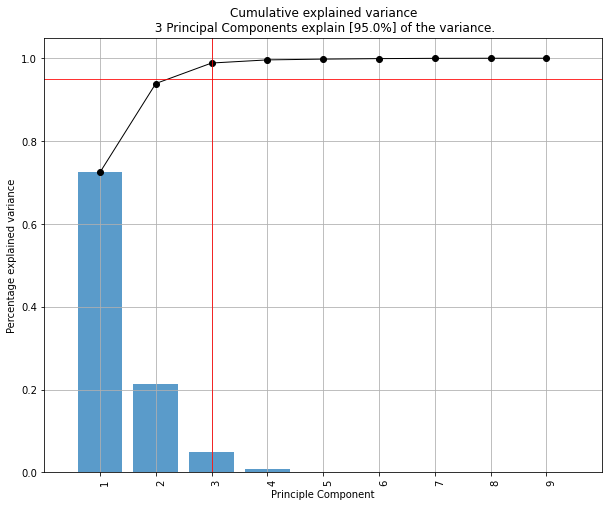An example of how principal component summarizes the variance in data. (Source: Stackoverflow)

## Applications

PCA is a handy data analysis tool. Therefore, PCA is useful in any field that uses a lot of data, including (but is not limited to) music, marketing, teaching, healthcare, and nuclear science. For example, a study from the Johannes Gutenberg Universitat-Mainz used PCA on data from a mouse experiment . They analyzed all the principal components to determine which variables corresponded to the most variance and were, therefore, the most important.

--

--